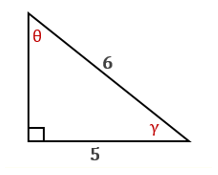Chapter 11.CT, Problem 9CTElementary Geometry For College St...

7th Edition
Alexander + 2 others
ISBN: 9781337614085

Solutions

Chapter
SectionElementary Geometry For College St...

7th Edition
Alexander + 2 others
ISBN: 9781337614085
Textbook Problem

In the drawing provided, find the measure of θ to the nearest degree.To determine

To find:

The measure the value of θ to the nearest degree.

Explanation

Procedure used:

In a right triangle, the sine ratio for an acute angle is the ratio oppsite sidehypotenuse.

Given:

The drawing as below.

Calculation:

From the diagram, hypotenuse = 6, opposite side = 5 and the angle is θ

Still sussing out bartleby?

Check out a sample textbook solution.

See a sample solution

The Solution to Your Study Problems

Bartleby provides explanations to thousands of textbook problems written by our experts, many with advanced degrees!

Get Started

Let f(x) = 1x+1 and g(x) = x2 + 1. Find the rules for (a) f + g, (b) fg (c) f t, and (d) g f.

Applied Calculus for the Managerial, Life, and Social Sciences: A Brief Approach

In Exercises 516, evaluate the given quantity. log22

Finite Mathematics and Applied Calculus (MindTap Course List)

Fill in each blank: 168ft=in.

Elementary Technical Mathematics